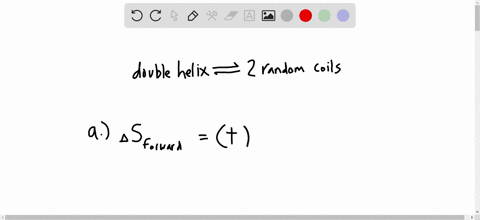Enroll in one of our FREE online STEM bootcamps. Join today and start acing your classes!View Bootcamps### When heated, the DNA double helix separates into …

04:32University of North Carolina at Chapel Hill
Problem 105

# A chemical reaction, such as HI forming from its elements, can reach equilibrium at many temperatures. In contrast, a phase change, such as ice melting, is in equilibrium at a given pressure and temperature. Each of the graphs below depicts $G_{\mathrm{sys}}$ vs. extent of change. (a) Which graph depicts how $G_{\mathrm{sys}}$ changes for the formation of HI? Explain. (b) Which graph depicts how $G_{\mathrm{sys}}$ changes as ice melts at 18C and 1 atm? Explain.

Check back soon!

## Discussion

You must be signed in to discuss.

## Video Transcript

So for the falling balance chemical equation, we want to look at two hypotheticals. So for the first we want to look at what happens if we keep a constant temperature for the system. What? We changed the volume. We're gonna look at a at A P versus one over the grant and then for the second part here, we don't wonder what would happen if we keep a constant pressure, but then changed the temperature. So we're gonna look at a volume versus temperature graph here. So, looking first for pressure versus one over volume for an ideal gas, we would see a straight line. This is thanks to what we know is Boyle's law, which is that pressure is proportional to one over the volume. We're looking at one over the volume here and the pressure on the Y axis here. But when we look at a system we know in terms of increasing one over V, we do not have the same number of moles starting out between the products and the reactions. So we're actually going to see a deviation in the system make that a little clearer at low one over V values. But when we look at V vs T volume versus temperature. That means we're putting volume on the Y axis in temperature on the X axis. Again, an ideal gas. Be a straight line. However, we know based on this envelope, you value here that since it is positive, we're adding we're gonna add heat to the reactant sign. So when we increased temperature were increasing, the heat added, which means we're going to shift the reaction to the right as to mature increases, which means that the volume is going to decrease. So we're going to see a change where the system figures off when the ideal gas constant is a straight line.

#### You're viewing a similar answer. To request the exact answer, fill out the form below:

Our educator team will work on creating an answer for you in the next 6 hours.